Anzeige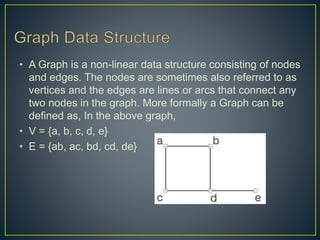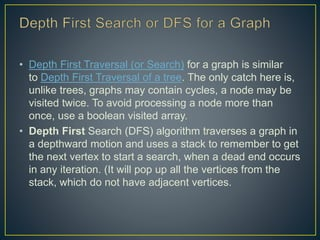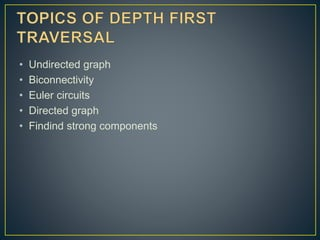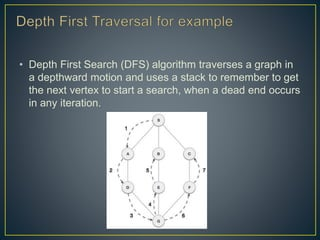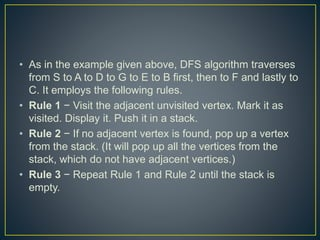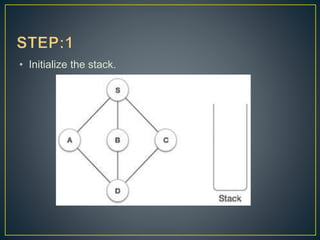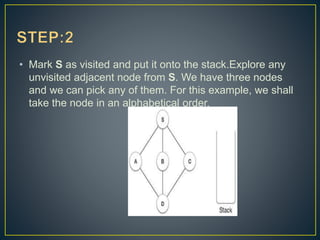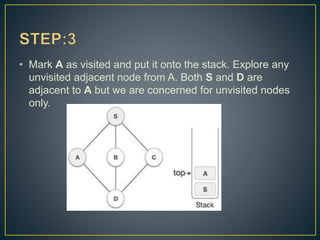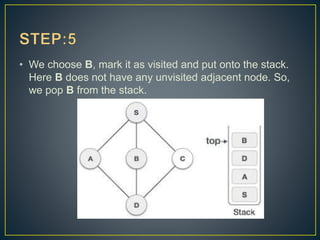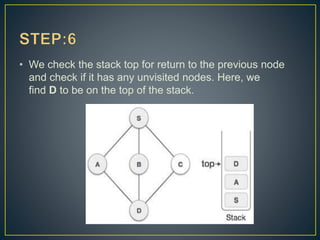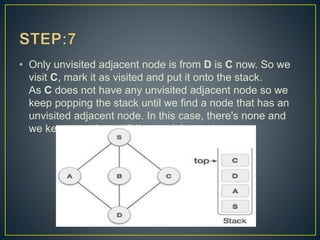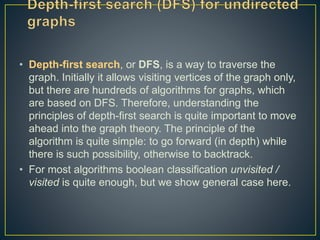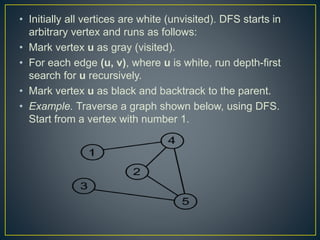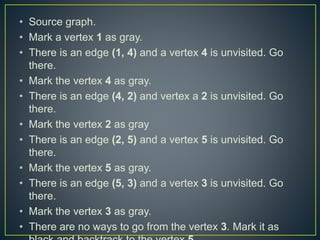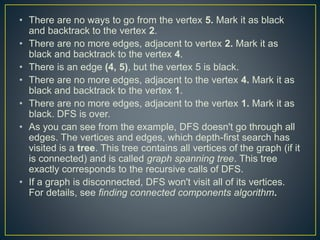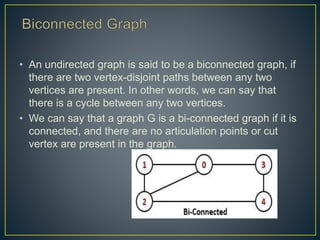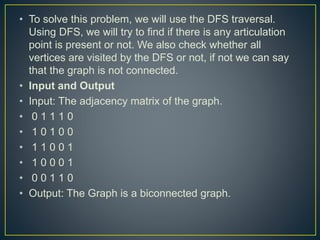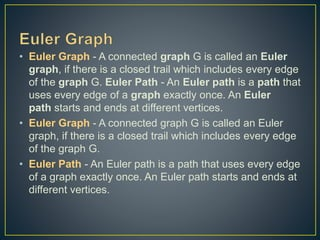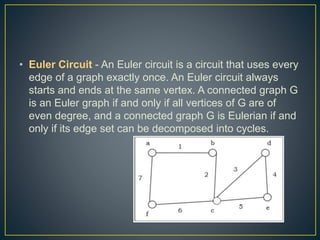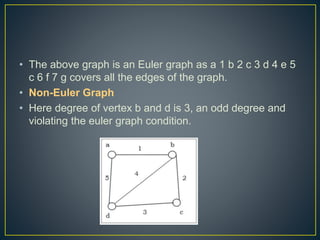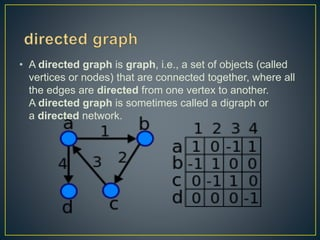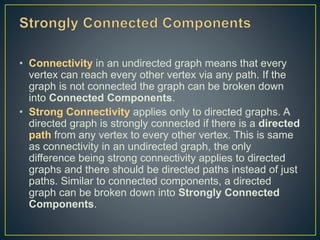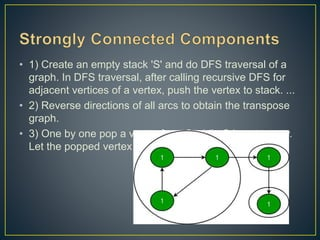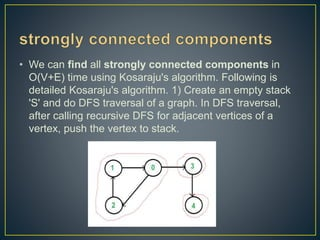1 von 27
Anzeige

### Depth first traversal(data structure algorithms)

1. By: A.Bhuvaneshwari,M.SC(cs)
2. • A Graph is a non-linear data structure consisting of nodes and edges. The nodes are sometimes also referred to as vertices and the edges are lines or arcs that connect any two nodes in the graph. More formally a Graph can be defined as, In the above graph, • V = {a, b, c, d, e} • E = {ab, ac, bd, cd, de}
3. • Depth First Traversal (or Search) for a graph is similar to Depth First Traversal of a tree. The only catch here is, unlike trees, graphs may contain cycles, a node may be visited twice. To avoid processing a node more than once, use a boolean visited array. • Depth First Search (DFS) algorithm traverses a graph in a depthward motion and uses a stack to remember to get the next vertex to start a search, when a dead end occurs in any iteration. (It will pop up all the vertices from the stack, which do not have adjacent vertices.
4. • Undirected graph • Biconnectivity • Euler circuits • Directed graph • Findind strong components
5. • Depth First Search (DFS) algorithm traverses a graph in a depthward motion and uses a stack to remember to get the next vertex to start a search, when a dead end occurs in any iteration.
6. • As in the example given above, DFS algorithm traverses from S to A to D to G to E to B first, then to F and lastly to C. It employs the following rules. • Rule 1 − Visit the adjacent unvisited vertex. Mark it as visited. Display it. Push it in a stack. • Rule 2 − If no adjacent vertex is found, pop up a vertex from the stack. (It will pop up all the vertices from the stack, which do not have adjacent vertices.) • Rule 3 − Repeat Rule 1 and Rule 2 until the stack is empty.
7. • Initialize the stack.
8. • Mark S as visited and put it onto the stack.Explore any unvisited adjacent node from S. We have three nodes and we can pick any of them. For this example, we shall take the node in an alphabetical order.
9. • Mark A as visited and put it onto the stack. Explore any unvisited adjacent node from A. Both S and D are adjacent to A but we are concerned for unvisited nodes only.
10. • Visit D and mark it as visited and put onto the stack. Here, we have B and C nodes, which are adjacent to D and both are unvisited. However, we shall again choose in an alphabetical order.
11. • We choose B, mark it as visited and put onto the stack. Here B does not have any unvisited adjacent node. So, we pop B from the stack.
12. • We check the stack top for return to the previous node and check if it has any unvisited nodes. Here, we find D to be on the top of the stack.
13. • Only unvisited adjacent node is from D is C now. So we visit C, mark it as visited and put it onto the stack. As C does not have any unvisited adjacent node so we keep popping the stack until we find a node that has an unvisited adjacent node. In this case, there's none and we keep popping until the stack is empty.
14. • Depth-first search, or DFS, is a way to traverse the graph. Initially it allows visiting vertices of the graph only, but there are hundreds of algorithms for graphs, which are based on DFS. Therefore, understanding the principles of depth-first search is quite important to move ahead into the graph theory. The principle of the algorithm is quite simple: to go forward (in depth) while there is such possibility, otherwise to backtrack. • For most algorithms boolean classification unvisited / visited is quite enough, but we show general case here.
15. • Initially all vertices are white (unvisited). DFS starts in arbitrary vertex and runs as follows: • Mark vertex u as gray (visited). • For each edge (u, v), where u is white, run depth-first search for u recursively. • Mark vertex u as black and backtrack to the parent. • Example. Traverse a graph shown below, using DFS. Start from a vertex with number 1.
16. • Source graph. • Mark a vertex 1 as gray. • There is an edge (1, 4) and a vertex 4 is unvisited. Go there. • Mark the vertex 4 as gray. • There is an edge (4, 2) and vertex a 2 is unvisited. Go there. • Mark the vertex 2 as gray • There is an edge (2, 5) and a vertex 5 is unvisited. Go there. • Mark the vertex 5 as gray. • There is an edge (5, 3) and a vertex 3 is unvisited. Go there. • Mark the vertex 3 as gray. • There are no ways to go from the vertex 3. Mark it as
17. • There are no ways to go from the vertex 5. Mark it as black and backtrack to the vertex 2. • There are no more edges, adjacent to vertex 2. Mark it as black and backtrack to the vertex 4. • There is an edge (4, 5), but the vertex 5 is black. • There are no more edges, adjacent to the vertex 4. Mark it as black and backtrack to the vertex 1. • There are no more edges, adjacent to the vertex 1. Mark it as black. DFS is over. • As you can see from the example, DFS doesn't go through all edges. The vertices and edges, which depth-first search has visited is a tree. This tree contains all vertices of the graph (if it is connected) and is called graph spanning tree. This tree exactly corresponds to the recursive calls of DFS. • If a graph is disconnected, DFS won't visit all of its vertices. For details, see finding connected components algorithm.
18. • An undirected graph is said to be a biconnected graph, if there are two vertex-disjoint paths between any two vertices are present. In other words, we can say that there is a cycle between any two vertices. • We can say that a graph G is a bi-connected graph if it is connected, and there are no articulation points or cut vertex are present in the graph.
19. • To solve this problem, we will use the DFS traversal. Using DFS, we will try to find if there is any articulation point is present or not. We also check whether all vertices are visited by the DFS or not, if not we can say that the graph is not connected. • Input and Output • Input: The adjacency matrix of the graph. • 0 1 1 1 0 • 1 0 1 0 0 • 1 1 0 0 1 • 1 0 0 0 1 • 0 0 1 1 0 • Output: The Graph is a biconnected graph.
20. • Euler Graph - A connected graph G is called an Euler graph, if there is a closed trail which includes every edge of the graph G. Euler Path - An Euler path is a path that uses every edge of a graph exactly once. An Euler path starts and ends at different vertices. • Euler Graph - A connected graph G is called an Euler graph, if there is a closed trail which includes every edge of the graph G. • Euler Path - An Euler path is a path that uses every edge of a graph exactly once. An Euler path starts and ends at different vertices.
21. • Euler Circuit - An Euler circuit is a circuit that uses every edge of a graph exactly once. An Euler circuit always starts and ends at the same vertex. A connected graph G is an Euler graph if and only if all vertices of G are of even degree, and a connected graph G is Eulerian if and only if its edge set can be decomposed into cycles.
22. • The above graph is an Euler graph as a 1 b 2 c 3 d 4 e 5 c 6 f 7 g covers all the edges of the graph. • Non-Euler Graph • Here degree of vertex b and d is 3, an odd degree and violating the euler graph condition.
23. • A directed graph is graph, i.e., a set of objects (called vertices or nodes) that are connected together, where all the edges are directed from one vertex to another. A directed graph is sometimes called a digraph or a directed network.
24. • Connectivity in an undirected graph means that every vertex can reach every other vertex via any path. If the graph is not connected the graph can be broken down into Connected Components. • Strong Connectivity applies only to directed graphs. A directed graph is strongly connected if there is a directed path from any vertex to every other vertex. This is same as connectivity in an undirected graph, the only difference being strong connectivity applies to directed graphs and there should be directed paths instead of just paths. Similar to connected components, a directed graph can be broken down into Strongly Connected Components.
25. • 1) Create an empty stack 'S' and do DFS traversal of a graph. In DFS traversal, after calling recursive DFS for adjacent vertices of a vertex, push the vertex to stack. ... • 2) Reverse directions of all arcs to obtain the transpose graph. • 3) One by one pop a vertex from S while S is not empty. Let the popped vertex be 'v'.
26. • We can find all strongly connected components in O(V+E) time using Kosaraju's algorithm. Following is detailed Kosaraju's algorithm. 1) Create an empty stack 'S' and do DFS traversal of a graph. In DFS traversal, after calling recursive DFS for adjacent vertices of a vertex, push the vertex to stack.
Anzeige